# Python Program to Make Calculator

In this article, I've created some programs in Python, to make a simple calculator. Here are the list of calculator programs in Python:

• Calculator Program using while loop and if-else
• Using user-defined Function
• Using Class and Object

## Calculator Program using while Loop and if-else

This program makes a simple calculator in Python that performs four basic mathematical operations such as add, subtract, multiply, and divide two numbers entered by user. Here I've provided 5 options to user, the fifth option is to exit.

```while True:
print("2. Subtraction")
print("3. Multiplication")
print("4. Division")
print("5. Exit")
print("Enter Your Choice (1-5): ", end="")
ch = int(input())
if ch>=1 and ch<=4:
print("\nEnter Two Numbers: ", end="")
numOne = float(input())
numTwo = float(input())
if ch==1:
res = numOne + numTwo
print("\nResult =", res)
elif ch==2:
res = numOne - numTwo
print("\nResult =", res)
elif ch==3:
res = numOne * numTwo
print("\nResult =", res)
elif ch==4:
res = numOne / numTwo
print("\nResult =", res)
elif ch==5:
break
else:
print("\nInvalid Input!..Try Again!")
print("------------------------")```

Here is the initial output produced by this Python program of simple calculator:Now supply the input. For example type 1 as choice, and press `ENTER` key, here is the output you'll see: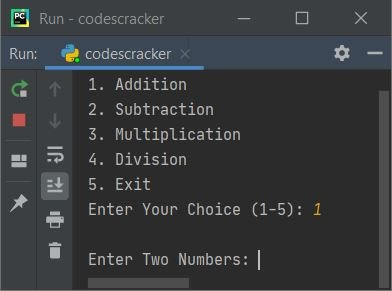Now enter any two numbers say 32 as first, press `ENTER` and 44 as second number, again press `ENTER`. Here is the output you'll see, that shows the result and again options gets displayed to operator further: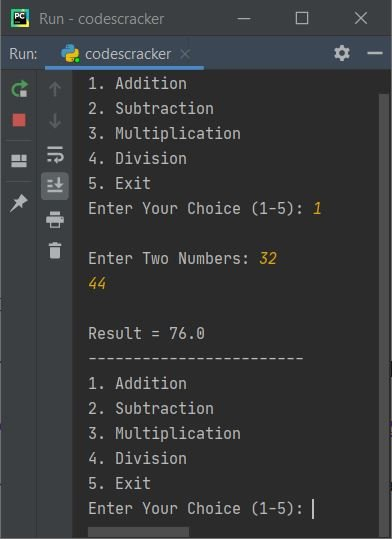To exit, type 5 as choice and press `ENTER`. The program execution gets terminated like shown in the snapshot given below: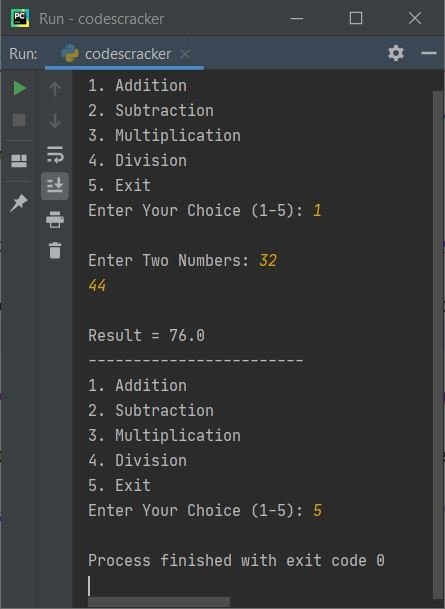The dry run of above program goes like:

• Since I've given True (boolean) as a condition of while loop, therefore program flow directly goes inside the loop without evaluating anything (condition), because the condition already written as True
• Inside the loop, I've provided 5 options. The first four options is for mathematical operations, whereas the fifth option is to exit from the loop and terminate the program
• Now through input(), the option (1-5) entered by user gets received and initialized to ch variable. For example, if user enters 1 as option, then ch=1
• The condition (first condition of if) ch>=1 or 1>=1 evaluates to be true, therefore the second condition gets evaluated, that is the condition ch<=4 or ch<=4 also evaluates to be true, therefore program flow goes inside this if's body
• Inside if's body, I've received two numbers and initialized to two variables namely numOne and numTwo respectively. For example, if user enters 32 and 44 as first and second numbers. Then numOne=32 and numTwo=44
• Now the condition (of next if) ch==1 or 1==1 evaluates to be true, therefore program flow goes inside this if's body and numOne+numTwo or 32+44 or 76 gets initialized to res. So res=76
• And the value of res gets printed as output
• Since if's condition evaluates to be true, therefore no any upcoming elif or else's statement(s) gets executed
• So using the last statement, I've printed some dashes (-------)
• Now again 5 choices gets displayed, and user has to enter his/her choice again to operate further. This process continues, since I've used True as condition of while loop, that always evaluates to be true
• The only way to exit from the loop, is when user enters 5 as choice, so that the condition of last elif, that is ch==5 or 5==5 evaluates to be true, and using break keyword, the execution of while loop gets ended

#### Modified Version of Previous Program

This program is the modified version of previous program. This program uses try-except to handle invalid inputs. That is, when user enters any invalid input like c, # as number, then program raises (prints) error message and continue asking to enter the valid one. Let's have a look:

```print("1. Addition")
print("2. Subtraction")
print("3. Multiplication")
print("4. Division")
print("5. Exit")
while True:
while True:
print("Enter Your Choice (1-5): ", end="")
try:
ch = int(input())
if ch>=1 and ch<=4:
print("\nEnter Two Numbers: ", end="")
numOne = float(input())
numTwo = float(input())

if ch==1:
print("\nResult =", numOne+numTwo)
elif ch==2:
print("\nResult =", numOne-numTwo)
elif ch==3:
print("\nResult =", numOne*numTwo)
elif ch==4:
print("\nResult =", numOne/numTwo)
elif ch==5:
break
else:
print("\nInvalid Input!..Try Again!")
print("------------------------")
except ValueError:
print("\nInvalid Input!..Try Again!")
print("------------------------")
continue
if ch==5:
break```

Here is its sample run with user input 3 as choice, then 2 and 5 as two numbers:As you can see from above sample run, the menu is displayed only once. Later on, I've only displayed the message that asks to enter the choice to continue the operation. Here is continued sample run with invalid and valid inputs: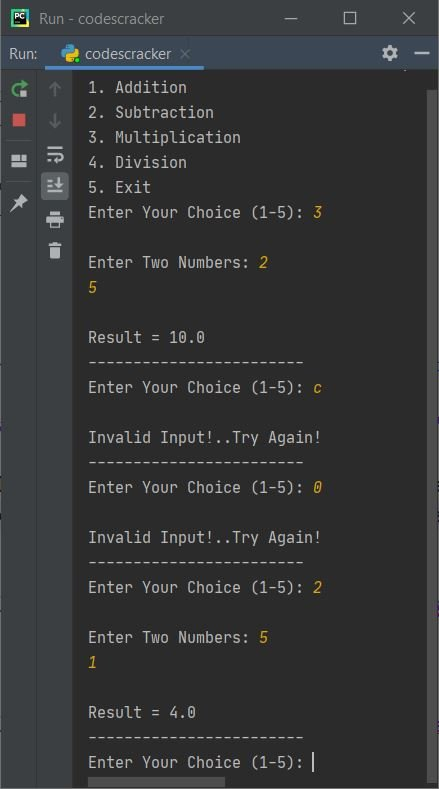The only way to exit the program, is by using 5 as choice.

Note - In above program, when user enters invalid input, then program flow goes to except ValueError's body and prints an error message. Then using continue keyword, program flow goes to the initial (first) statement of while loop's body to receive the input again inside try

## Calculator Program using Function

This program is created using four user-defined functions. All functions receives two arguments and returns the corresponding result.

```def add(a, b):
return a+b
def sub(a, b):
return a-b
def mul(a, b):
return a*b
def div(a, b):
return a/b

print("2. Subtraction")
print("3. Multiplication")
print("4. Division")
print("5. Exit")
while True:
while True:
print("Enter Your Choice (1-5): ", end="")
try:
ch = int(input())

if ch>=1 and ch<=4:
print("\nEnter Two Numbers: ", end="")
nOne = float(input())
nTwo = float(input())

if ch==1:
elif ch==2:
print("\nResult =", sub(nOne, nTwo))
elif ch==3:
print("\nResult =", mul(nOne, nTwo))
elif ch==4:
print("\nResult =", div(nOne, nTwo))
elif ch==5:
break
else:
print("\nInvalid Input!..Try Again!")
print("------------------------")

except ValueError:
print("\nInvalid Input!..Try Again!")
print("------------------------")
continue
if ch==5:
break```

This program produces same output as of previous program.

## Calculator Program using Class

This is the last calculator program in Python, created using class. To access member function of a class, an object is required. Therefore an object ob is created of a class named CodesCracker, of which I've to access member functions using dot (.) operator.

```class CodesCracker:
return a+b
def sub(self, a, b):
return a-b
def mul(self, a, b):
return a*b
def div(self, a, b):
return a/b

print("2. Subtract")
print("3. Multiply")
print("4. Divide")
print("5. Exit")

while True:
while True:
print("Enter Your Choice (1-5): ", end="")

try:
ch = int(input())

if ch>=1 and ch<=4:
print("\nEnter Two Numbers: ", end="")
nOne = float(input())
nTwo = float(input())
ob = CodesCracker()

if ch==1:
print("\n" +str(nOne)+ " + " +str(nTwo)+ " = " + str(ob.add(nOne, nTwo)))
elif ch==2:
print("\n" +str(nOne)+ " - " +str(nTwo)+ " = " + str(ob.sub(nOne, nTwo)))
elif ch==3:
print("\n" +str(nOne)+ " * " +str(nTwo)+ " = " + str(ob.mul(nOne, nTwo)))
elif ch==4:
print("\n" +str(nOne)+ " / " +str(nTwo)+ " = " + str(ob.div(nOne, nTwo)))
elif ch==5:
break
else:
print("\nInvalid Input!..Try Again!")
print("------------------------")

except ValueError:
print("\nInvalid Input!..Try Again!")
print("------------------------")
continue

if ch==5:
break```

Here is its sample run with some user inputs: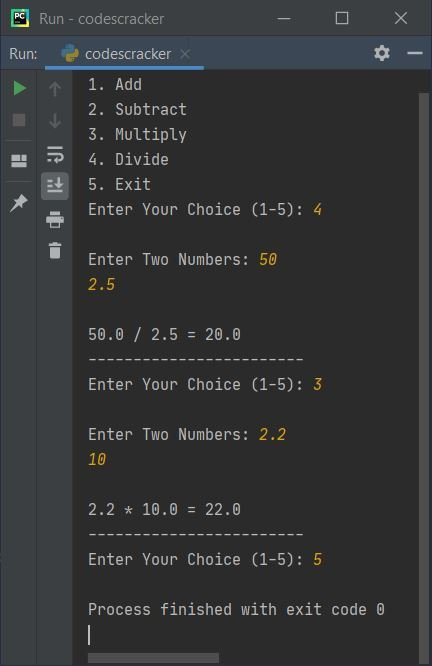#### Same Program in Other Languages

Python Online Test

« Previous Program Next Program »